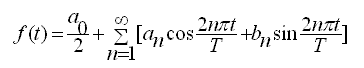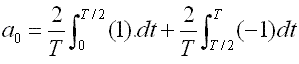# Fourier Series of Periodic Functions

Tutorials on Fourier series are presented. In the first part an example is used to show how Fourier coefficients are calculated and in a second part you may use an app to further explore Fourier series of the same function.

## Fourier Series and Coefficients

Fourier series may be used to represent periodic functions as a linear combination of sine and cosine functions. If f(t) is a periodic function of period T, then under certain conditions, its Fourier series is given by:where n = 1 , 2 , 3 , ... and T is the period of function f(t). an and bn are called Fourier coefficients and are given byExample 1
Find the Fourier series of the periodic function f(t) defined bySolution to Example 1
Coefficient a0 is given byCoefficients an is given byAnd coefficients bn is given byA computation of the above coefficients gives
$a_0 = 0$, $a_n = 0$ and $b_n = \dfrac{2}{n\pi} (1 - \cos (n \pi))$
Note that $\cos (n \pi)$ may be written as
$cos (n \pi) = (-1)^n$
and that bn = 0 whenever n is even.
The given function f(t) has the following Fourier series## Interactive Tutorial on Fourier Series

For numerical calculations purposes we cannot include an infinite number of terms in the series above, we therefore define function $f_N(t)$ with a limited number of terms $N$ as follows $f_N(t) = \sum_{n=1}^{N} \dfrac{2}{n\pi} (1-(-1)^n) \sin(\dfrac{2 n \pi t}{T})$ The app below may be used to explore the Fourier series of f(t) solved in example 1 above including a limited number of terms $N$ in the series and see how the graph of function $f_N(t)$ defined above becomes close to the graph of function f(t) as $N$ increases.

The default values are $N = 5$ and $T = 4$ and the series have one term which is a sinusoidal function of period T. Incease $N$ and compare the graph of the function obtained (in blue) to that of $f(t)$ (in red) defined in example.

 $N$ = 5 $T$ = 4
Change $N$ and $T$ and click this button

Hover the mousse cursor on the graph or plotted point to read the coordinates.